# Zero Exponents

The exponent of a number says how many times to use the number in a multiplication. However a zero exponent creates a bit of a puzzle because you cannot multiply a number zero times. However, we can derive the rule from the exponent rules for division. Any number divided by itself is equal to one. For example. If we look at the rules for division which says that when you divide you subtract exponents we get the following: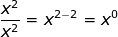and since we already know thatit follows that x° = 1 . This means that any number (except zero) raised to the zero power is 1.

Example 1: 3° = 1

Example 2: 12° = 1

Example 3: 101° = 1

Example 4: 31,456,236,719 ° = 1

Example 5: (-5)° = 1

Example 6: 0° =undefined ??? This is similar to dividing by zero. It is undefined.

Hmmm...Do you see an emerging pattern? We can write the rule as a° =1. You can remember it as any number (except zero) to the zero power is 1.

The most common mistake is to assume that the answer is zero. If you hesitate use your calculator to verify.

How does this apply when you are simplifying variable expressions that have an exponent of 0?

Example 7: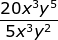Rewrite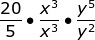Subtract Exponents when you divide   4 •x3-3 y5-2

Simplify  4 •x° • y3

Zero exponent rule  4 • 1 • y3

Final result  4y3 therefore the x3canceled out

Let's think through this logically. We know that any number divided by itself is equal to one.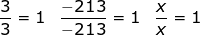So it makes sense that any number to the zero power is one when we look at: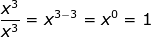Related Links: Math Exponents Exponents and Powers The Laws of Exponents Integers as Exponents Evaluate Exponents Evaluate integers with exponents Positive and Negative Integer Exponents Rational (Fractional) Exponents

To link to this Zero Exponents page, copy the following code to your site: